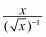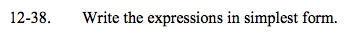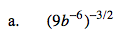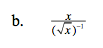### Home > A2C > Chapter 12 > Lesson 12.1.2 > Problem12-38

12-38.
1. Write the expressions in simplest form. Homework Help ✎

1. (9b−6)−3/2

2.Remember the properties of exponents.

$\small\left(9^{-\frac{3}{2}}\right)\left(\left(b^{-6}\right)^{-\frac{3}{2}}\right)$

$\small\left(3^{-3}\right)\left(b^{\frac{18}{2}}\right)$

$\frac{b^9}{27}$$\small x^{\frac{3}{2}}\text{ or }x\sqrt{x}$